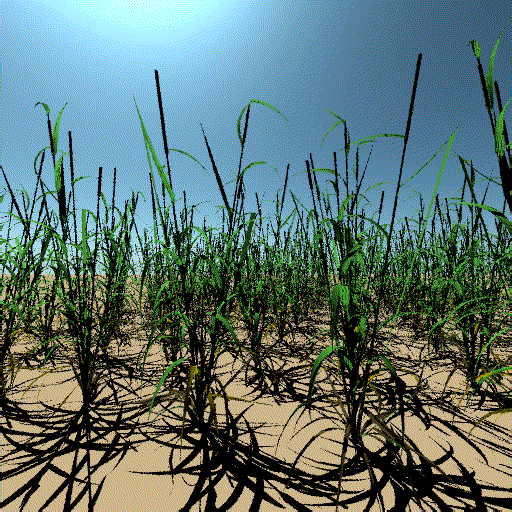# Introduction to radiative transfer modelling¶

## J Gómez-Dans (UCL & NCEO)¶

j.gomez-dans@ucl.ac.uk## Introduction to modeling lectures¶

1. Motivation
2. Modelling leaf optical properties
3. Modelling canopies in the optical domain

### Motivation¶

• Model: formulation of physical principles that produce signals you measure.
• Models are simplifications of reality, and thus are not reality
• We need to know strengths AND weaknesses of models
• In EO, we are interested in processes that describe how photon-atmosphere-canopy interactions $\Rightarrow$ radiative transfer theory (RT)
• Some interesting quotations (H/T Mat Disney@UCL

“All models are wrong but some are useful” – George Box

“The purpose of models is not to fit the data but to sharpen the questions” – Samuel Karlin

“No one trusts a model except the person who wrote it. Everyone trusts an observation except the person who made it.” – Anon.

### Some uses of physcal models¶

• Inform data collection
• Observng System Observation Experiments (OSSEs)
• Satellite mission simulators
• Consistent treatment of data from different instruments
• Can treat spectral, angular and spatial acquisition characteristics
• Data pre-processing and conditioning
• Consistent use of data from e.g. microwave, optical and thermal domain.
• Parameter retrieval
• The model can be used to interpret observations in terms of model input parameters
• Interpolation/Extrapolation
• Extend observations
• Understand sensitivity of observation• modelling entails assumptions
• You need to understand the assumptions
• ... and assess whether they are acceptable for your application!

### Physical models and EO¶

• Typically want to monitor processes over vast areas, different time scales...
• EO is an indirect measurement, so data we acquire needs to be interpreted
• Eg: we measure radiance from a sensor
• ... but you said you were interested in delimiting land use!
• ... bit you said you were interested in assessing fire danger!
• Data interpretation can be done in two ways:
• Empirical models: measure magnitude of interest $\leadsto$ pairing with EO data $\leadsto$ finding a statistical relationship
• Mechanistic models: by considering the processes that produce the observations, and using this understanding to quantify variables of interest.

### An example of an empirical model¶*From Ouyang et al (2012)

### An example of a mechanistic model¶### Assumptions!¶

• In both cases, there are assumptions:
• An empirical model is typically derived for a particular
• Location
• Time
• Sensor
• A mechanistic model makes assumptions on
• processes included and ignored
• simplifications of the processes
• Physical models explicitly enconde basic properties of system
• Energy conservation
• Reciprocity

### Model error¶

• Observations have experimental error (e.g. errorbars!)
• Model error encodes the price that the model inadequacy in predicting reality
• It is usually very hard to assess formally
• ... and thus often disregarded